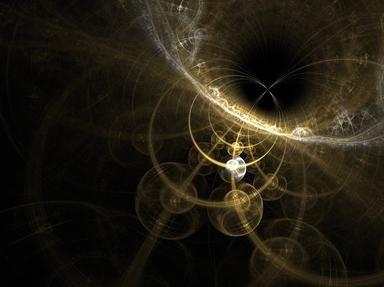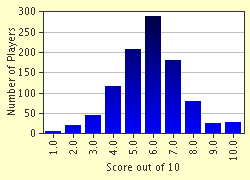# Infinity Trivia Quiz

### Infinity is really, really big. But what else can we say about it? Is it a number? Is it possible for something to be bigger than infinity? Take this quiz and find out!

A multiple-choice quiz by riotgrrl. Estimated time: 5 mins.

Author
riotgrrl
Time
5 mins
Type
Multiple Choice
Quiz #
86,067
Updated
Dec 03 21
# Qns
10
Difficulty
Tough
Avg Score
6 / 10
Plays
17801
Awards
Editor's Choice
Last 3 plays: Guest 173 (8/10), Guest 173 (7/10), Guest 24 (3/10).
This quiz has 2 formats: you can play it as a or as shown below.
Scroll down to the bottom for the answer key.
1. The mathematical symbol for infinity looks like which character turned on its side? Hint

The letter 'S'
The number '7'
The number '8'
The letter 'P'

#### NEXT>

2. Which Dutch artist used many mathematical concepts, including infinity, in his work? Hint

Bosch
Pablo Picasso
M C Escher
Rembrandt

#### NEXT>

3. As there are an infinite number of whole numbers, how can the number of even numbers between one and infinity always be described? Hint

None
Infinity divided by two
Infinity
Square root of infinity

#### NEXT>

4. If you want to move from A to B, you must first move half the distance from A to B. Then you must travel half the remaining distance. Then half of that, and so on, doing infinitely many moves. So, assuming you are moving at a constant speed, how is it possible to ever get anywhere? Hint

It is theoretically impossible
Because each move takes proportionally less time
Because you move in paces, not infinitely small distances
Because infinitely small distances are an impossibility

#### NEXT>

5. One googol is 1 with 100 zeroes after it (10^100). One with one googol zeroes after it (10^[10^100]) is termed a 'googolplex'. So is a googolplex closer in value to infinity than one is?

Yes
No

#### NEXT>

6. It can be shown that the total number of real numbers (that is, numbers including fractions, pi, etc.) is different to the total number of whole numbers (1,2,3,etc.). So how is the second of these, the total number of whole numbers, described? Hint

A
Alpha0
Aleph0
A0

#### NEXT>

7. "The Paradoxes of the Infinite" was written in 1851 by which man? Hint

George Bernard Shaw
Jean-Paul Sartre
Bernard Bolzano
Gottfried Leibnitz

#### NEXT>

8. So what is infinite? Is time infinite? Hint

Maybe
No, definitely not
Yes, definitely
Infinity and time are unrelated concepts

#### NEXT>

9. A black hole is, some theories predict, infinitely dense. So can one ever be detected? Hint

Yes, if we look straight at it
Yes, from its effects on nearby things
Yes, but only if we could ever reach one
No, they can only exist in theory

#### NEXT>

10. Which great mathematician's work do modern concepts of infinity stem from? Hint

Leonardo Fibonacci
Albert Einstein
Georg Cantor
Leonard Euler

 (Optional) Create a Free FunTrivia ID to save the points you are about to earn:Select a User ID:Choose a Password:Your Email:

Quiz Answer Key and Fun Facts
1. The mathematical symbol for infinity looks like which character turned on its side?

The Romans sometimes used this symbol to represent 1000, a big number, and this led a mathematician named John Wallis to suggest in 1650 that it should be used as the symbol of infinity. It stuck.
2. Which Dutch artist used many mathematical concepts, including infinity, in his work?

Escher is well known for his 'impossible' drawings, including staircases which go nowhere and hands drawing each other. Although not a trained mathematician, Escher is accepted to have had an intuitive understanding of the discipline.
3. As there are an infinite number of whole numbers, how can the number of even numbers between one and infinity always be described?

There are also infinitely many. You could write any whole number with an even number twice as large alongside. This is called a one-to-one correspondence, and it is important because for any list of numbers with this one-to-one relationship to whole numbers, it can be seen there are infinitely many (and this infinity has the same value).
4. If you want to move from A to B, you must first move half the distance from A to B. Then you must travel half the remaining distance. Then half of that, and so on, doing infinitely many moves. So, assuming you are moving at a constant speed, how is it possible to ever get anywhere?

Answer: Because each move takes proportionally less time

This is known as Zeno's Paradox after Zeno of Elea, a fifth century BC philosopher who proposed many paradoxes on the subject of infinity. Fortunately, this particular one can be refuted, otherwise nothing would ever get done!
5. One googol is 1 with 100 zeroes after it (10^100). One with one googol zeroes after it (10^[10^100]) is termed a 'googolplex'. So is a googolplex closer in value to infinity than one is?

No, it isn't. A googolplex is a very, very big number. But it is different in concept to infinity, which is not a number. The remaining numbers can still be put in a one-to-one correspondance with the 'set' of all whole numbers. So you can't be any closer to infinity, as you've still got just as many numbers left to go.
6. It can be shown that the total number of real numbers (that is, numbers including fractions, pi, etc.) is different to the total number of whole numbers (1,2,3,etc.). So how is the second of these, the total number of whole numbers, described?

Aleph is the first letter of the Hebrew alphabet, and it is used with a subscript to describe these two kinds of infinity. Other infinities are described Aleph1, Aleph2, and so on. The total number of real numbers might be equal to Aleph1 - but all that is known for sure is that it is at least Aleph1 - it might be more!
7. "The Paradoxes of the Infinite" was written in 1851 by which man?

Born in Prague, Bolzano numbered mathematician and philosopher among his several talents. He wrote in German, with "The Paradoxes of the Infinite" concentrating primarily on set theory.
8. So what is infinite? Is time infinite?

Scientists are uncertain as to whether time is infinite. It all depends on whether the total mass of the universe is sufficient to drag it all back together in a reversal of the Big Bang, which would effectively end time, or whether the universe will continue expanding, which would mean an infinite amount of time lies ahead.
9. A black hole is, some theories predict, infinitely dense. So can one ever be detected?

Answer: Yes, from its effects on nearby things

Yes, the immense gravitational fields surrounding black holes deflect electromagnetic radiation (such as light) passing nearby. This is the only obvious way, as looking straight at one would not reveal it (hence the term 'black'). Anything getting close enough to a black hole to detect it directly would be unable to send any details back to us, as they would be drawn in to the black hole.
10. Which great mathematician's work do modern concepts of infinity stem from?

The Russian Georg Cantor (1845 - 1918) revolutionised maths by overcoming resistance to investigating infinity. His concepts were so novel that he famously said "I see it, but I don't believe it".
Source: Author riotgrrl

This quiz was reviewed by FunTrivia editor crisw before going online.
Any errors found in FunTrivia content are routinely corrected through our feedback system.
Most Recent Scores
Jan 29 2023 : Guest 173: 8/10
Jan 25 2023 : Guest 173: 7/10
Jan 24 2023 : Guest 24: 3/10
Jan 24 2023 : OldTowneMal: 3/10
Jan 24 2023 : Guest 76: 5/10
Jan 22 2023 : Guest 81: 8/10
Jan 22 2023 : Guest 102: 3/10
Jan 19 2023 : GuyCognito: 6/10
Jan 19 2023 : Guest 51: 3/10

Score Distribution